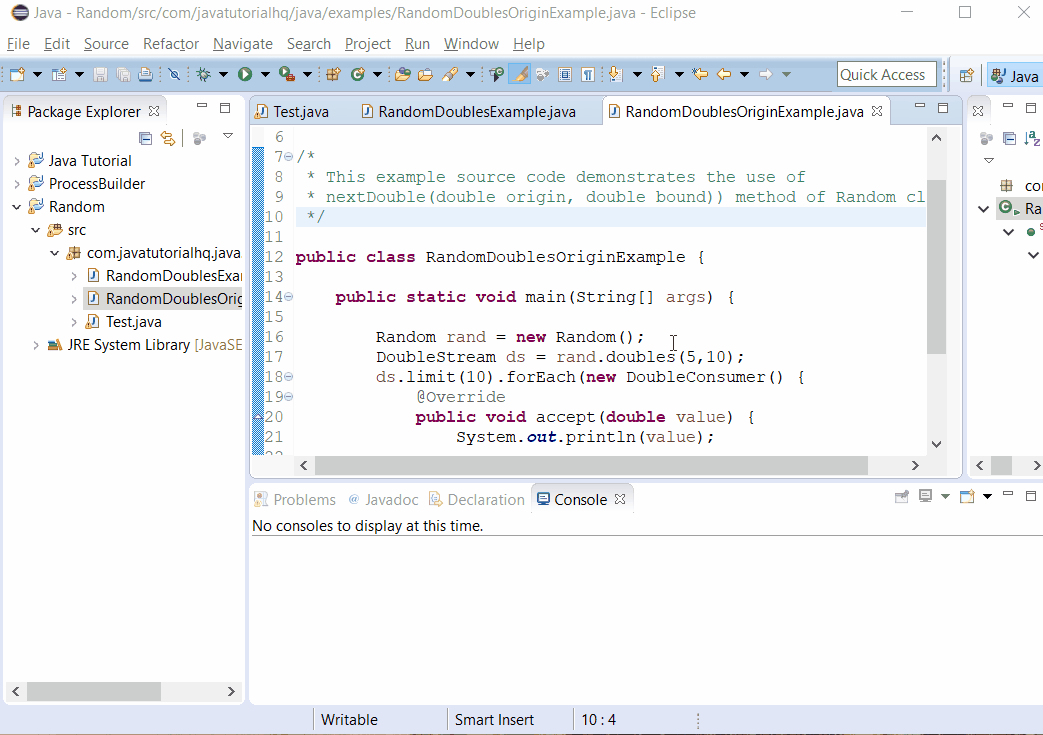# Java Random doubles(double randomNumberOrigin, double randomNumberBound) method example

HQ » Java Tutorial » java.util » Random » doubles(double randomNumberOrigin, double randomNumberBound)
Java Random doubles(double randomNumberOrigin, double randomNumberBound) method example 2018-02-28T13:34:48+00:00

## Description

The doubles(double randomNumberOrigin, double randomNumberBound) method of Random class is an overloaded method of doubles(). Basically this method returns an effectively unlimited stream of pseudorandom double values, each conforming to the given origin (inclusive) and bound (exclusive).

## Method Syntax

public DoubleStream doubles(double randomNumberOrigin, double randomNumberBound)

## Method Argument

Data Type Parameter Description
double randomNumberOrigin the origin (inclusive) of each random value
double randomNumberBound the bound (exclusive) of each random value

## Method Returns

The doubles() method of Random class returns a stream of pseudorandom double values, each with the given origin (inclusive) and bound (exclusive).

Java 1.8

## Discussion

The method doubles(double randomNumberOrigin, double randomNumberBound) is an overloaded method of doubles() wherein on this method requires two input which is randomNumberOrigin and randomNumberBound.

By calling this method a pseudorandom double value is generated as if it’s the result of calling the following method with the origin and bound:

## Java Random doubles() Example

Below is a simple java example on the usage of doubles() method of Random class.

Basically on the above example, we put a limit on the number of values inside the Stream by calling the method limit and then by using the foreach method of DoubleStream class 10 random double values from range 6 to 10 has been printed out.

## Sample Output

Below is the sample output when you run the above example.## References

Singapore

#### Our Goal

JavaTutorialHQ aims to to be The Ultimate Guide on Java with hundreds of examples from basic to advance Topics.

By continuing to use the site, you agree to the use of cookies. more information

The cookie settings on this website are set to "allow cookies" to give you the best browsing experience possible. If you continue to use this website without changing your cookie settings or you click "Accept" below then you are consenting to this.

Close